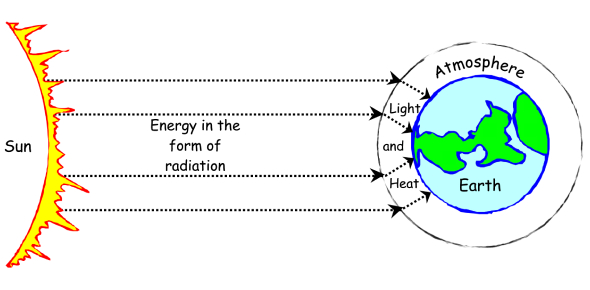# Quiz: Forms Of Energy!

14 Questions | Attempts: 11747
ShareSettingsWhy do people drink that morning coffee everyday before going to work? Why do we need to eat? Why do our dogs need to be walked multiple times per day? All of these things are related to the concept of energy – the get-up-and-go our bodies need in order to function in numerous ways. Energy can come in many different forms and we’re going to be testing your knowledge on them all in this quiz!

• 1.
Energy comes in all different forms.
• A.

True

• B.

False

• 2.
Energy is not in everything.
• A.

True

• B.

False

• 3.
Energy is constantly changing from one form to another:
• A.

Law of energy

• B.

Energy conservation

• C.

Refraction

• D.

Reflection

• 4.
Energy that is stored or the energy of position.
• A.

Kinetic

• B.

Potential

• 5.
The energy of motion.
• A.

Kinetic

• B.

Potential

• 6.
We only have on light source.
• A.

True

• B.

False

• 7.
Light travels in a straight line until it strikes an object or travels from on median to another.
• A.

True

• B.

False

• 8.
What is the ability to do work?
• A.

Light

• B.

Gravity

• C.

Energy conservation

• D.

Energy

• 9.
How many forms of energy are there?
• A.

3

• B.

8

• C.

2

• D.

9

• 10.
Matter can be changed into what?
• A.

Energy

• B.

Gravity

• C.

Motion

• D.

Light

• 11.
Energy can be changed into what?
• A.

Light

• B.

Motion

• C.

Gravity

• D.

Matter

• 12.
What are the two categories that forms if energy can be placed into?
• A.

Potential and Light

• B.

Light and Kinetic

• C.

Potential and Kinetic

• D.

Light and Gravity

• 13.
Can energy change its form?
• A.

Yes

• B.

No

• 14.
When a material gets wet and absorbs water, what is effectively changed?
• A.

Potential energy

• B.

Material's index of fraction

• C.

Kinetic energy

• D.

The light source

## Related TopicsBack to top
×

Wait!
Here's an interesting quiz for you.